## Momentum Conservation - Complete Toolkit

### Objectives

1. To apply Newton’s second and third law to collisions in order to compare the force and the accelerations of the two colliding objects.
2. To understand that there are four quantities that are always the same for the two colliding objects: force, time, impulse and momentum change.
3. To express an understanding of the law of momentum conservation by comparing momentum changes and total system momentum for a collision.
4. To use the law of momentum conservation to analyze a collision problem and to determine an unknown quantity.
5. To use the law of momentum conservation as a guide to proportional reasoning in order to predict the post-collision velocity of a colliding object in an inelastic collision.
6. To apply the law of momentum conservation to the analysis of explosions.

### Interactive Simulations

1. Collision CartsThe Collision Carts Interactive provides a virtual collision track for colliding or exploding carts. The mass of the two carts and their pre-collision velocities can be varied. Inelastic and elastic collisions or explosions can be conducted. Post-collision velocities are displayed. This HTML5 Interactive works on smart phones, tablets such as the iPad, Chromebooks, laptops and desktops. It's the perfect solution for 1-to-1 physics classrooms.

2. PhET Collision LabUse an air hockey table to investigate simple collisions in one dimension or more complex collisions. You can experiment with the number of discs, masses, and initial conditions.  Students will construct momentum vector representations of “before and after” collisions, explain why energy is not conserved in some collisions, and understand what “elasticity” means when applied to collisions. Using the sim, students collect experimental data to form conclusions about momentum conservation. For a great accompanying class activity, see the link below.

PhET Collision Lab: Student Experiment

3. Open Source Physics: Collision Between Two Pendulums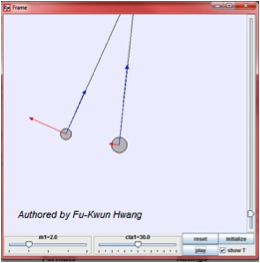This Java model lets users simulate a collision between two pendulums. The simulation assumes elastic collision between the two objects. Students can adjust mass and initial angle for one pendulum. Click “T” to view tension. No mathematics is introduced; rather, the activity is intended to promote understanding of how momentum is conserved in isolated systems and the effect of mass on momentum conservation.

4. Exploding Carts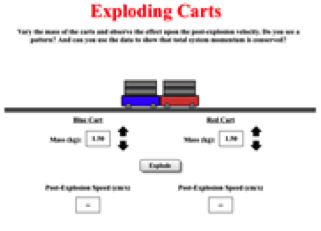The Exploding Carts Interactive animates several explosions between two carts on a no-friction track. The explosions differ in terms of the relative mass of the two carts. Post-collisions speeds of the two carts are shown. Trials can be performed with a varying ratio of mass for the two carts. While any user could easily discover the physics of explosions by simply opening and exploring, The Physics Classroom has provided a guided activity sheet that leads students to understanding the connection between the mass ratios of the two carts and the ratio of post-explosion velocities. The exercise excels at promoting students' science reasoning skills.

### Video and Animations

1. Veritasium: Bullet Block Experiment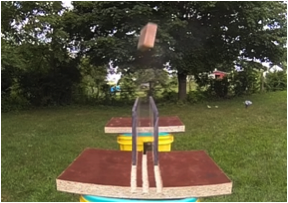Take the Bullet Block challenge! YouTube's Veritasium channel, created by and starring physicist Derek Muller, presents an interesting scenario. A bullet is fired into the precise center of a block of wood and its motion recorded with high-speed photography. The block is moved so the second bullet strikes the right corner of the wood block. Will the second impact cause the block to go A) Higher, B) The same height, or C) Lower than the first block. The correct answer might surprise you. It all has to do with conservation of momentum!  Link to the explanation:  Explanation of Bullet Block Experiment

2. WatchKnowLearn.org – Mythbusters Car Crash Force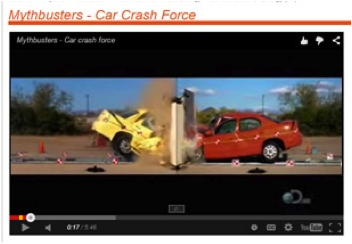This 6-minute video summarizes the Mythbusters show "Car Crash Force", with the best of the slo-mo video. We watch two cars of identical design and mass being crashed into a wall -- one at 50 mph and one at 100 mph. The damage to the faster-moving car is a great way to envision why the kinetic energy equation works for car crash (KE = 1/2 mass x velocity squared). So.....what if we again take two cars of identical design & mass and crash them into each other? Mythbusters' Jamie Hyneman predicts incorrectly. How will your students predictions go? Will they consider conservation of momentum?  Editor’s Note: See item directly below for the related “Aftershow” that explains the science.

3. Mythbusters: Crash Force AftershowWithout this 4-minute "aftershow" explanation, the Mythbusters Car Crash Force could be shoved in the "interesting trivia" file. This video connects the crash to the physics. In the Car Crash Force video, two cars of identical design and mass traveling at 50 mph are crashed head-on into each other. The video of the damage looks the same as that of one car (same design/mass) crashing head-on into a wall at 50 mph. Mythbuster's Jamie Hyneman had incorrectly predicted that two cars hitting each other at 50 mph would "be the same as one car hitting a wall at 100 mph". But WHY was he wrong? And what if the two cars were different masses? This video delves into those questions.

4. Physlet Physics: Momentum ProblemsIf you use clickers or student response systems, this set of 14 Physlet problems would be an ideal addition to your toolkit. The problems are animation-based, with multiple choice answers provided. Teachers can obtain answer keys by registering on the Physlet website.

5. Physlet Physics:  Understanding Conservation LawsThis mini-collection from Physlet Physics features 7 Java-based animations that delve into conservation of momentum in both elastic and inelastic collisions. Two and three-object systems are featured, as well as an explosive collision. Bonus-- each animation has a pdf worksheet created specifically for classroom use.

6. Richard Hammond’s Blast Wave in Slow MotionFANTASTIC videography showcases two explosions to clearly depict the propagation of a blast wave that accompanies an explosion. The video clip is a great class warm-up, but doesn't cover the physics related to collisions, so you might want to point your students to this article by Gary Settles, professor of mechanical engineering at Penn State's Gas Dynamics Laboratory:  Scientific American: High Speed Imaging & the Physics of Explosions Written in layman's terms, it's a great synopsis of how collisions at the molecular scale drive the creation of a thin wavefront that can cause incredible destruction. Until recently, we couldn't see these waves closely, but super high-speed videography has changed all that.

7. Circus Physics:  Linear MomentumThis video-based resource explores linear momentum through a platform system consisting of two trapeze artists. The momentum of the system is the sum of the momenta of all objects in it. The video is crafted to help students understand how momentum is conserved as one trapeze artist jumps off the platform and launches into the air. This resource includes a teacher's guide with tips on how to incorporate the video into instruction, discussion questions, and accompanying classroom activities.

### Labs and Investigations

1. The Physics Classroom, The Laboratory, Before and After

Students observe elastic and inelastic collisions and make comparisons of before- and after velocities of the colliding objects.

2. The Physics Classroom, The Laboratory, Action-Reaction

Students quantitatively analyze an explosion between two carts of known mass to determine the change in velocity and momentum.

3. The Physics Classroom, The Laboratory, Sand Balloon

Students apply momentum conservation to an inelastic collision between a sand-filled balloon and a moving cart to determine the mass of the balloon

4. The Physics Classroom, The Laboratory, Inelastic Collision Analysis

Students use motion detectors to analyze an inelastic collision and to compare before- and after-collision values for the total system momentum.

5. The Physics Classroom, The Laboratory, Elastic Collision Analysis

Students use motion detectors to analyze an elastic collision and to compare before- and after-collision values for the total system momentum.

6. The Physics Classroom, The Laboratory, What's Cooking?

Students measure the after-explosion velocity of a home-made tennis ball cannon in order to determine the launch velocity of the tennis ball.

### Interactive Homework Problems Based on PER  (Physics Education Research)

1. Illinois PER Interactive Problems:  Spring-Loaded Collision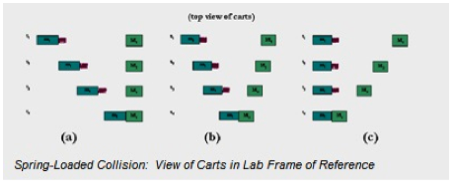Here’s another great concept-builder from physics education researcher Gary Gladding. This interactive problem presents a completely elastic collision between two carts.  The author takes students step-by-step through conceptual exploration and strategic analysis before they tackle any math. The exercise is carefully crafted to help students visualize the physical situation from two frames of reference:  the lab frame and the center-of-mass frame (CM).  By setting up the problem to show the relationship between the lab frame and CM frame, students will discover that the problem-solving can be made considerably easier.

2. Illinois PER Interactive Problems:  Ballistic Pendulum Extension Activity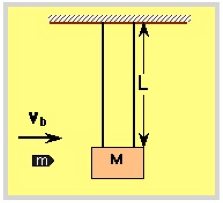This is an interactive homework problem for introductory physics students involving conservation of energy and conservation of momentum.  A bullet is fired, embedding itself in a resting pendulum suspended vertically by massless strings.  Given mass of the bullet and pendulum, plus initial velocity of the bullet, how high will the pendulum rise after the collision?  Editor’s Note: We like this problem because the author deftly guides students to discover that momentum problems often require consideration of energy conservation. The math is pretty simple, even if you haven’t covered Energy yet.

### Minds On Physics Internet Modules:

The Minds On Physics Internet Modules are a collection of interactive questioning modules that target a student’s conceptual understanding. Each question is accompanied by detailed help that addresses the various components of the question.
1. Momentum and Collisions, Ass’t MC4 -  Force, Impulse and Momentum Change
2. Momentum and Collisions, Ass’t MC5 -  Momentum Conservation
3. Momentum and Collisions, Ass’t MC6 -  Explosion-Like Impulses
4. Momentum and Collisions, Ass’t MC7 -  Momentum Conservation
5. Momentum and Collisions, Ass’t MC8 -  Problem-Solving - Inelastic Collisions
6. Momentum and Collisions, Ass’t MC9 -  Problem-Solving - Elastic Collisions
7. Momentum and Collisions, Ass’t MC10 -  Momentum and Proportional Reasoning

### Concept Building Exercises:

1. The Curriculum Corner, Momentum and Collisions, Action-Reaction and Momentum Conservation
2. The Curriculum Corner, Momentum and Collisions, Collision Analysis
3. The Curriculum Corner, Momentum and Collisions, Momentum Problem-Solving
4. The Curriculum Corner, Momentum and Collisions, Momentum Conservation as a Guide to Thinking

### Problem-Solving Exercises:

1. The Calculator Pad, Momentum and Collisions, Problems #12 - #30

### Science Reasoning Activities:

1. The Science Reasoning Center – Momentum and Collisions - Collisions

### Common Misconceptions:

1. Velocity is Conserved

Somehow students develop the incorrect notion that velocity is conserved in a collision. Their belief is that if an object moving at 4 m/s collides and sticks to an object that is at rest, then the after collision velocity of each individual object is 2 m/s. This would provide a total velocity of 2 m/s, enough to convince such students that momentum was conserved. Object mass plays no role in their analysis. Similarly, if an object moving at 6 m/s catches up and collides and sticks to an object moving at 2 m/s, then the after-collision velocity of each object is 4 m/s. Once more, the analysis ignores the importance of mass and is totally incorrect under all conditions with the exception of the condition that each object has an identical mass. Emphasize the fact that momentum is the conserved quantity.

### Extension Activities:

1. Open Source Physics:  Ejs Hard Disk Collision Model Interactive Computer Model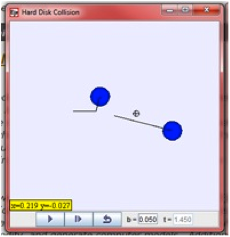In introducing collisions in two dimensions, simpler can be better. We like this simulation because it confines a collision problem to a very simple scenario of two balls that collide in an isolated system. The collision is viewed from the reference frame that is moving with the center of mass of the two balls. This means students don't have to deal with an algebraic nightmare to do the math that will quantify both momentum and conservation of energy. Center of mass is shown as a cross in the simulation. Click the cross to see x and y coordinates.....then the math becomes pretty easy.

2. Open Source Physics: Two Particle Elastic Collision Model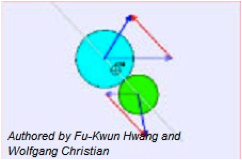For students ready to amp it up to 2-D collision scenarios, this model provides an ideal segue from the simpler simulations highlighted above.  Mass, position and velocity of each disk can be changed using sliders.  Both disks are draggable, allowing students to set up one-dimensional and two-dimensional collisions. A checkbox option will pause the simulation at the moment of collision, and then display arrows showing the momenta of each disk immediately before AND after the collision.  Red arrows depict the change in momentum resulting from the collision. The instructions explain how to set up equations to calculate both the total energy and total momentum of the system after the collision.

### Standards:

A. Next Generation Science Standards (NGSS)

Performance Expectations – Motion and Stability: Forces and Interactions
• HS-PS2-3    Apply scientific and engineering ideas to design, evaluate, and refine a device that minimizes the force on a macroscopic object during a collision.
• HS-PS2-2     Use mathematical representations to support the claim that the total momentum of a system of objects is conserved when there is no net force on the system.

Disciplinary Core Ideas
• HS-PS2.A.ii    Momentum is defined for a particular frame of reference; it is the mass times the velocity of the object.

Crosscutting Concepts

Systems and System Models – Crosscutting Concept #4
• When investigating or describing a system, the boundaries and initial conditions of the system need to be defined and their inputs and outputs analyzed and described using models.
• Models can be used to predict the behavior of a system, but these predictions have limited precision and reliability due to the assumptions and approximations inherent in models.
• Models (e.g., physical, mathematical, computer models) can be used to simulate systems and interactions – including energy, matter, and information flows – between and within systems at different scales.

Stability and Change – Crosscutting Concept #7
• Much of science deals with constructing explanations of how things change and how they remain stable.
• Systems can be designed for greater or lesser stability.
• Change and rates of change can be quantified and modeled over very short or very long periods of time. Some system changes are irreversible.

Science and Engineering Practices

Practice #1: Analyzing and Interpreting Data
• Analyze data using computational models in order to make valid and reliable scientific claims.

Practice #2: Developing and Using Models
• Develop and use a model based on evidence to illustrate the relationships between systems or between components of a system.
• Use a model to provide mechanistic accounts of phenomena.

Practice #3: Planning and Carrying Out Investigations
• Plan and conduct an investigation individually and collaboratively to produce data to serve as the basis for evidence, and in the design: decide on types, how much, and accuracy of data needed to produce reliable measurements and consider limitations on the precision of the data (e.g., number of trials, cost, risk, time), and refine the design accordingly.

Practice #6: Obtaining, Evaluating, and Communicating Information
• Communicate scientific ideas (e.g. about phenomena and/or the process of development and the design and performance of a proposed process or system) in multiple formats (including orally, graphically, textually, and mathematically).

Practice #8: Using Mathematics and Computational Thinking
• Use mathematical representations of phenomena to describe explanations.
• Create a computational model or simulation of a phenomenon, designed device, process, or system.
• Use mathematical models and/or computer simulations to predict interactions between systems.